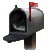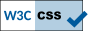R.E.D. Server: Examples & demonstration
Execution of the Ante_R.E.D. 2.0 and R.E.D. IV programs

E. Vanquelef
Université de Picardie - Jules Verne, Amiens

P. Cieplak
Sanford Burnham Prebys Medical Discovery Institute, La Jolla, CA

Université de Picardie - Jules Verne, Amiens

This tutorial demonstrates how the Ante_R.E.D. 2.0 and R.E.D.-IV (June 2010) programs interfaced with R.E.D. Server can be used to (i) derive RESP or ESP charges, and to (ii) generate force field libraries for a large panel of molecules and molecular fragments. Molecules taken from the Tutorial -I- as well as new structures are presented. The goal of this tutorial is not to provide extensive explanations on charge derivation and force field library building, which can be found in Tutorial -I-, but rather to describe examples of P2N files generated and used by R.E.D. Server to build force field library(ies) with embedded RESP or ESP charges in the Tripos mol2 file format.

This tutorial might also be useful for users interested in running the R.E.D.-III.x Tools in a standalone mode (i. e. without using R.E.D. Server). However, it is important to underline that some molecular fragments and force field topology databases can only be generated using the R.E.D.-IV program. Thus, some strategies presented below might not be compatible with the R.E.D.-III.x Tools. The two logos "R.E.D.-III.x compatible" and "R.E.D.-III.x incompatible" are used throughout this tutorial to guide the reader.

It is important to underline that the Ante_R.E.D. and R.E.D. programs do not differentiate a "standard" from a "non-standard" residue. Consequently, both cases are not differentiated in this tutorial, and can be alternatively employed by the user.   .

Finally, we would to suggest to R.E.D.-III.x and R.E.D. Server users to always run Ante_R.E.D. 2.0 before executing R.E.D. Indeed, errors dealing with charge equivalencing are one of the most common problem encountered when one derives RESP or ESP charge values for a new molecule or a molecular fragment. Using Ante_R.E.D. 2.0 ensures a rigorous definition of chemical equivalencing and consequently correct charge equivalencing.

The list of corrections applied to this tutorial after its first release can be obtained here.

This tutorial has been updated in agreement with the new features incorporated in Ante_R.E.D. 2.0, R.E.D. IV June 2010 and R.E.D. Server 2.0.

Description of R.E.D. Server 2.0
Examples: R.E.D. Server inputs & final outputs
-I- General information
-II- Charge derivation and force field library building for a single molecule
-II.1- Organic molecule
-II.2- Amino-acid dipeptide
-II.3- Deoxyribonucleoside
-II.4- Metal complex
-III- Charge derivation and force field library building for multiple molecules
-III.1- Ten organic molecules
-III.2- Two amino acid dipeptides
-III.3- Four ribonucleosides
-III.4- -III.1-, -III.2- & -III.3- in a single R.E.D.-IV run ?
-IV- Charge derivation and force field library building for a single molecular fragment
-IV.1- Central fragment of an amino acid
-IV.2- (+)NH3-terminal fragment of an amino acid
-IV.3- (-)OOC-terminal fragment of an amino acid
-IV.4- Central fragment of a nucleotide
-IV.5- 5'-terminal fragment of a nucleotide
-IV.6- 3'-terminal fragment of a nucleotide
-IV.7- Molecular fragment of a metal complex
-V- Force Field Topology DataBase building
-V.1- Definition of a "Force Field Topology DataBase"
-V.2- Set of amino acid fragments
Set of amino acid fragments automatically generated from a single dipeptide
-V.3- Set of nucleotide fragments
Set of nucleotide fragments automatically generated from a single nucleoside
-V.4- Set of glycoconjugate fragments
-VI- All together in a single R.E.D.-IV run?

Description of R.E.D. Server 2.0

The global procedure for deriving RESP or ESP charge values and building force field library(ies) for new molecules and/or new molecular fragments is summarized in Figure 1: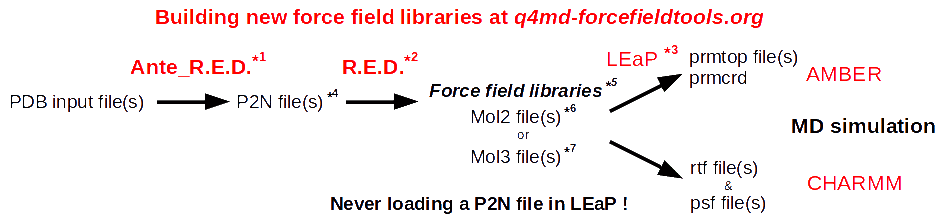*1  Ante_R.E.D. 1.x from the R.E.D. III.x tools or Ante_R.E.D. 2.0 at R.E.D. Server *5  Important features of a force field library: *2  R.E.D. III.x from the R.E.D. III.x tools or R.E.D. IV at R.E.D. Server - Chemically equivalent atoms bear the same charge value *3  tLEaP or xLEaP (handle force fields & force field libraries): - Two atoms belonging to a residue cannot share the same name - Two atoms sharing the same name in a given residue are not recognized by LEaP ! *6  Description of the Tripos mol2 file format here and here - Force field atom types are added by using a LEaP script (see an example here) *7  Description of the mol3 file format here *4  A P2N file is a PDB file with two columns of atom names: Main characteristics (more information here, and an example here): - 1st column of atom names reflecting chemical equivalencing            (chemically equivalent atoms bear the same atom name; yellow column here) - 2nd column of atom names reflecting IUPAC naming conventions            (two atoms belonging to a residue cannot share the same name; red column here)
Figure 1

R.E.D. Server is open to all users, and registration to use R.E.D. Server is not mandatory. R.E.D. Server provides the software and hardware (i. e. a cluster of computers) required for the derivation of RESP and ESP atomic charges and the building of force field libraries for computational biologists involved in AMBER, CHARMM, GLYCAM & OPLS force field based biological studies (Scheme 1). R.E.D. Server interfaces the latest stable versions of the Ante_R.E.D. 2.0 and R.E.D.-IV programs developed by the q4md force field tools team, and provides access to the binaries for the latest version of the Gaussian (2003 and 2009), GAMESS-US, or the Firefly programs, and for the RESP program. More generally, the latest developments in term of RESP and ESP charge derivation carried out by the q4md force field tools team will be available through R.E.D. Server. The release of these new developments/features as well as their description are available at the R.E.D. Server news web page.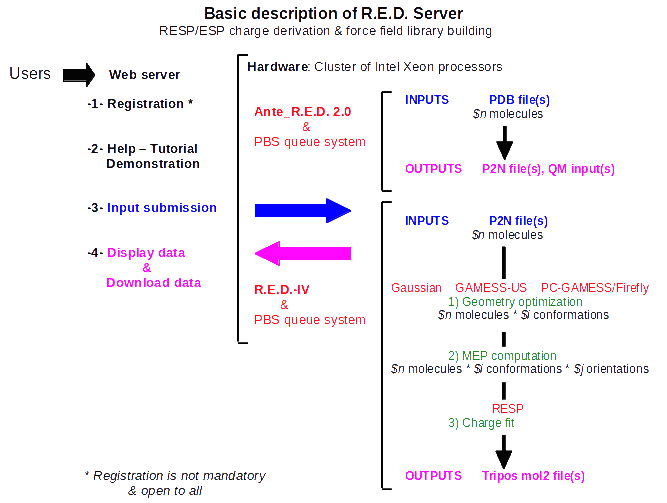Scheme 1

Please, read the R.E.D. Server FAQ, which provide information about R.E.D. Server and its different tools. A demonstration is also available from the Demonstration (Demo) service at the R.E.D. Server home page.

If a user needs help on using R.E.D. Server, a general public help is provided with the q4md-forcefieldtools mailing list. Any researcher can participate in this mailing list by answering and/or sending queries at q4md-fft@q4md-forcefieldtools.org after registration at sympa@q4md-forcefieldtools.org. To register simply send an email to sympa@q4md-forcefieldtools.org with "subscribe q4md-fft" in the email subject or body (to un-subscribe just send "unsubscribe q4md-fft"). Archives of the q4md-fft mailing list are public. A private assistance is also available for registered users from the Assistance service available at the R.E.D. Server Home page. We are registered in the AMBER and CCL mailing lists, and we will answer queries about the q4md force field tools in these two mailing lists as well.

Examples: R.E.D. Server inputs & final outputs

-I- General information

The P2N file format is the input file format used by the R.E.D. program. This file format derives from the Protein Data Bank file format. The P2N file format contains (i) two columns of atoms names, one used in the automatic generation of the input(s) of the RESP program, while the other one is involved in the conservation of international atom name conventions required in any force field library, (ii) information regarding molecular orientation, conformation and molecular topology (iii) the IUPAC name of the molecule, (iv) the spin multiplicity as well as (v) the molecule total charge. A characteristic example of a P2N file containing two molecular conformations is available in Tutorial -I-.

The Ante_R.E.D. program can be used to transform a PDB file into a P2N file. However, a user must always check the P2N files generated by Ante_R.E.D. Indeed, in our opinion several modifications in the P2N file format can only be carried out by a human, and using a "black box" approach is not always appropriate. Thus, a user has to understand the three simple rules required in the definition of the column of atom names used in chemical equivalencing and in the automatic generation of the RESP program input(s). These rules are the basis for an efficient charge fitting step with a low RRMS value. The control of the molecular orientation of any optimized geometry is another key aspect developed in the R.E.D. program. It takes a particular importance to derive reproducible RESP and ESP charge values. Checking the atom connectivities is also crucial for getting a correct molecular topology in a force field library. Finally, the definition of the atom names as well as the residue(s) is essential, when one wants to develop a new force field library. Reading the "Mini HowTo" available in Tutorial -I- should provide a step by step procedure for charge derivation and force field library building.

R.E.D. Server 2.0 interfaces the latest version of the Ante_R.E.D. and R.E.D. programs (i. e. Ante_R.E.D. version 2.0 and R.E.D. IV version June 2010, so far). These two programs present many new functionalities compared to the R.E.D. III.x Tools. New features developed in Ante_R.E.D. 2.0 are the resolution of molecular topology (with recontruction of the topology for bad input geometries), the determination of rigorous chemical equivalencing (used in charge equivalencing), the development of various approaches for atom reordering as well as the definition of atom names and residues (i. e. two atoms in a residue belonging to a force field library cannot share the same name). R.E.D. IV June 2010 presents many new features as well. This includes the reinforcement of charge reproducibility, the recalculation of Cartesian coordinates for molecular fragments originating from the fusion between two molecules, the building of complex force field topology databases for any type of bio-organic and bio-inorganic molecules, the generation of all-atom force field libraries or united-carbon force field libraries as well as the development of a statistic module allowing charge value comparisons (i. e. a first attempt for validating charge values).

-II- Charge derivation and force field library building for a single molecule

-II.1- Organic molecule       R.E.D.-III.x compatible

The first example concerns a small organic molecule: dimethylsulfoxide. This molecule adopts a single stable conformation, which can be located in space in many different orientations. Hence, a single conformation and two different molecular orientations are used during charge derivation leading to highly reproducible charge values.

1st step: Run the Ante_R.E.D. 1.4 program on your local workstation using the PDB input file available here, or upload this PDB input file to R.E.D. Server to execute Ante_R.E.D. 2.0.
2nd step: Compare the PDB input file and the P2N file generated by Ante_R.E.D. 1.4 to the P2N file generated by Ante_R.E.D. 2.0/R.E.D. Server, and rename the later one into Mol_red1.p2n.
Remark: Atom equivalencing for the two methyl groups of dimethylsulfoxide (reflected by the atom names in the first column of atom names in the P2N file) generated by Ante_R.E.D. 2.0 is correct, and does not need to be manually adapted. On the contrary, the user has still to modify the atom names of the dimethylsulfoxide methyl groups in the first column of atom names to make them chemically equivalent when using Ante_R.E.D. 1.4.
3rd step: Upload this Mol_red1.p2n file [with or without the corresponding geometry optimization output obtained by quantum mechanics (QM) calculations] to R.E.D. Server.
4th step: After the R.E.D.IV/R.E.D. Server job is completed, download the data generated by R.E.D. Server (a single P\$x.tar.bz2 file, where \$x is an internal R.E.D. Server job number) from the Download service available at the R.E.D. Server home page, or from the web address provided at the end of the input submission procedure. Among all the available files, load the Tripos mol2 file (name: Mol_m1-o1.mol2) as a force field library in the LEaP program, and/or visualize the structure and charge values in VMD, etc... This file is available in R.E.DD.B. in the project W-4. In this project, the RESP-A1A (or "RESP-A1" using R.E.D.-III.x) charge model was used to compute the charge set and to generate the force field library. The selection of the charge model among the ones in a list is done by the user during the input submission step in R.E.D. Server.

A complex example of chemical equivalencing was provided by Alan Wilter: the PDB input file, the P2N file generated by Ante_R.E.D. 2.0, and the corresponding Java applet are provided (in this example the P2N file generated by Ante_R.E.D. 1.4 is far more limited).

In the future, the integration of the Ante_R.E.D. and R.E.D. jobs described above into a single step will be implemented in R.E.D. Server.

-II.2- Amino acid dipeptide       R.E.D.-III.x compatible

The second example describes how to derive charges for the N-Acetyl-L-alanine-N'-methylamide dipeptide. In this example, the molecule is represented by three different molecular conformations: C5, C7ax and C7eq. Four different molecular orientations for each optimized conformation of this dipeptide are used in the charge derivation procedure leading to a 12-structures charge fit (three conformations * four molecular orientations).

1st step: Run the Ante_R.E.D.-1.4 program using the PDB input files (C5.pdb, C7ax.pdb and C7eq.pdb) corresponding to three conformations of N-Acetyl-L-alanine-N'-methylamide, or upload these PDB input files to R.E.D. Server to execute Ante_R.E.D. 2.0.
2nd step: Compare the P2N files generated by Ante_R.E.D. 1.4 (C5-out.p2n, C7ax-out.p2n and C7eq-out.p2n) generated by Ante_R.E.D. 1.4 to those generated by Ante_R.E.D. 2.0/R.E.D Server (C5-out.p2n, C7ax-out.p2n and C7eq-out.p2n).
Remark: As there is no error in the PDB input files and as chemical equivalencing is basic in the alanine dipeptide studied here there is no fundamental difference in the P2N files generated by the two Ante_R.E.D. versions. Then, one needs to merge these three P2N files into a single file, and rename the latter to Mol_red1.p2n. This Mol_red1.p2n file presents three sets of Cartesian coordinates in the same atom order that are used in charge derivation involving three conformations.
3rd step: Upload Mol_red1.p2n (with or without the corresponding geometry optimization outputs obtained by QM calculations and concatenated into a single file) to R.E.D. Server to execute R.E.D. IV.
4th step: After the R.E.D. Server job is completed, download the data generated by R.E.D. Server (a single P\$x.tar.bz2 file). Among all the available files, select one of the three Tripos mol2 files (names: Mol_m1-o1.mol2, Mol_m1-o2.mol2 or Mol_m1-o3.mol2; "-o\$i" being the conformation number) as these files contain identical charge values.

During the input submission step in R.E.D. Server select the charge model of your choice among the ones available in the list.

R.E.DD.B. contains several projects about the N-Acetyl-L-alanine-N'-methylamide dipeptide. The W-58 R.E.DD.B. project is an example of RESP charge derivation for this dipeptide involving 3 conformations * 10 molecular orientations.

-II.3- Deoxyribonucleoside       R.E.D.-III.x compatible

The third example demonstrates how to derive charges for the deoxyadenosine nucleoside. In this example, the molecule is represented by two different molecular conformations: C2'endo and C3'endo. Four different molecular orientations for each optimized conformation of this nucleoside are used in the charge derivation procedure leading to a two conformations * four molecular orientations charge fit.

1st step: Execute the Ante_R.E.D.-1.4 program using the PDB input files (C2endo.pdb and C3endo.pdb) corresponding to two conformations of deoxyadenosine, or upload these PDB input files to R.E.D. Server to execute Ante_R.E.D. 2.0.
2nd step: Compare the P2N files (C2endo-out.p2n and C3endo-out.p2n) generated by Ante_R.E.D. 1.4 to those generated by Ante_R.E.D. 2.0/R.E.D Server (C2endo-out.p2n and C3endo-out.p2n).
Remark: As there is no error in the PDB input files and as chemical equivalencing is basic in the nucleoside studied here there is no fundamental difference in the P2N files generated by the two Ante_R.E.D. versions. Then one needs to merge these two P2N files into a single file, and rename the latter to Mol_red1.p2n. This Mol_red1.p2n file presents two sets of Cartesian coordinates in the same atom order that are used in charge derivation involving two conformations.
3rd step: Upload Mol_red1.p2n (with or without the corresponding geometry optimization outputs obtained by QM calculations and concatenated into a single file) to R.E.D. Server to execute R.E.D. IV.
4th step: After the R.E.D. Server job is completed, download the data generated by R.E.D. Server (a single P\$x.tar.bz2 file). Among all the available files, select one of the two Tripos mol2 files (names: Mol_m1-o1.mol2 or Mol_m1-o2.mol2; "-o\$i" being the conformation number) as these files contain identical charge values.

During the input submission step in R.E.D. Server select the charge model of your choice among the ones available in the list.

R.E.DD.B. contains several projects on the deoxyadenosine nucleoside. The W-64 R.E.DD.B. project is an example of RESP charge derivation for this nucleoside involving 2 conformations * 12 molecular orientations.

-II.4- Metal complex       R.E.D.-III.x compatible

R.E.D. handles charge derivation for chemical elements up to Bromine (Z = 35 in the periodic table). It does not differentiate between a molecule that has a metal atom or the one that does not have it. A key aspect for building the the force field library for an organo-metallic complex is to provide in the Mol_red\$n.p2n input file the correct atom connectivities describing the bonds between the metal and the organic part of the molecule. A key point for the QM calculations is to correctly define the spin multiplicity of the complex in agreement with its total charge in the Mol_red\$n.p2n input file.

The fourth example deals with Cobalt(III)_hexammine. In this example, the molecule is represented by a single conformation, and two different molecular orientations are used in the charge derivation procedure.

1st step: Run the Ante_R.E.D.-1.4 program using the PDB input file available here, or upload this PDB input files to R.E.D. Server to execute Ante_R.E.D. 2.0
2nd step: Compare the PDB input file and the P2N file generated by Ante_R.E.D. 1.4 to the P2N file generated by Ante_R.E.D. 2.0/R.E.D. Server, and rename the later one into Mol_red1.p2n.
Remark: Atom equivalencing for the six ammonias of Cobalt(III) hexammine (reflected by the atom names in the first column of atom names in the P2N file) generated by Ante_R.E.D. 2.0 is correct, and does not need to be manually adapted. On the contrary, the user has still to modify the atom names of the Cobalt(III)_hexammine ammonias in the first column of atom names to make them chemically equivalent when using Ante_R.E.D. 1.4 [Cobalt(III) hexammine presents a spin multiplicity of 1 and a total charge of +3; the cobalt atom has six bonds with six nitrogen atoms].
3rd step: Upload the Mol_red1.p2n file (with or without the corresponding geometry optimization outputs obtained by QM calculations and concatenated into single file) to R.E.D. Server to execute R.E.D. IV,
4th step: After the R.E.D. Server job is completed, download the data generated by R.E.D. Server (a single P\$x.tar.bz2 file). Among all the available files, select the Tripos mol2 file (name: Mol_m1-o1.mol2).

During the input submission step in R.E.D. Server select the charge model of your choice among the ones available in the list. In some cases, a specific charge model might be required to generate correct charge values for an organo-metallic complex. New charge models incorporated in R.E.D.-IV will be released in R.E.D. Server in a near future (see the R.E.D. Server news web page for more information).

-III- Charge derivation and force field library building for multiple molecules

R.E.D. Server handles in a single R.E.D.-IV run the charge derivation and the force field library building for a set of defined molecules.

-III.1- Ten organic molecules       R.E.D.-III.x compatible

In this new example ten Mol_red\$n.p2n files (\$n = 1 up to 10) corresponding to ten organic solvents are prepared and uploaded to R.E.D. Server (with or without the corresponding geometry optimization outputs obtained by QM calculations). A single conformation and two to four molecular orientations are used for each optimized conformation in the charge derivation procedure. This set of ten molecules is also used in the Demo service available at the R.E.D. Server home page. However, the PDB input files used is this tutorial are different to those presented in the demontration. Indeed, while there is no error in the PDB files involved in this tutorial errors have been volontary incomporated in the PDB input files of the demonstration to highlight the new features incorporated in Ante_R.E.D. 2.0.

The execution of the Ante_R.E.D. program can be automated using the following Multi-Ante-RED.csh csh script:

#!/bin/csh
#
touch Ante_RED.log
foreach PDBFILE (*.pdb)
echo \$PDBFILE
perl Ante_RED-X.X.pl \$PDBFILE >> Ante_RED.log
end
echo "All done..."

Table 1 lists the different PDB input files, P2N files generated by Ante_R.E.D. 1.4 and Ante_R.E.D. 2.0 as well as the ten Mol_red\$n.p2n files to be uploaded to R.E.D. Server to execute R.E.D. IV.

 Solvent PDB input files (*1) P2N files (*2) P2N files (*3) Mol_red\$n.p2n Dimethylsulfoxide DMSO.pdb DMSO-out.p2n DMSO-out.p2n Mol_red1.p2n Ethanol EtOH.pdb EtOH-out.p2n No fundamental difference Mol_red2.p2n Trifluoroethanol TFE.pdb TFE-out.p2n TFE-out.p2n Mol_red3.p2n Methanol MeOH.pdb MeOH-out.p2n No fundamental difference Mol_red4.p2n Acetone Acetone.pdb Acetone-out.p2n Acetone-out.p2n Mol_red5.p2n Acetic acid AcOH.pdb AcOH-out.p2n No fundamental difference Mol_red6.p2n Acetonitrile MeCN.pdb MeCN-out.p2n No fundamental difference Mol_red7.p2n Benzene Benz.pdb Benz-out.p2n Benz-out.p2n Mol_red8.p2n Toluene Tol.pdb Tol-out.p2n Tol-out.p2n Mol_red9.p2n Chloroform CHL.pdb CHL-out.p2n CHL-out.p2n Mol_red10.p2n
(*1) There is no error in the PDB input files - see the Demo for comparison; (*2) generated by Ante_R.E.D. 1.4; (*3) generated by Ante_R.E.D. 2.0/R.E.D. Server.
Table 1

Select the charge model of your choice among the ones available in the list. Downloaded data contain ten "Mol_m\$n" directories corresponding to the charge derivation of the ten molecules taken individually and a "Mol_MM" directory corresponding to the charge derivation of these molecules taken all together. In the present example, Tripos mol2 files can be obtained either from each individual "Mol_m\$n" directory (names = Mol_m\$n-o\$i.mol2) or from the "Mol_MM" directory (names: mm\$n-o\$i.mol2, \$n = molecule number, \$i = conformation number; in the present case \$n = 1 to 10 and \$i = 1 independently of the molecule). The following PDF file contains the description of the different files generated by R.E.D. Server for this ten-molecules job.

You might decide to choose different options than those presented in this example for the conformations of ethanol or trifluoroethanol and/or for the control of the molecular orientation of each optimized geometry.

R.E.DD.B. contains several projects dealing with these solvent molecules (see the W-46, W-47, W-48 & W-49 R.E.DD.B. projects which only differ by the charge model used during charge derivation).

-III.2- Two amino acid dipeptides       R.E.D.-III.x compatible

In this new example two Mol_red\$n.p2n files (\$n = 2) corresponding to the N-Acetyl-2-aminoisobutyric_acid-N'-methylamide (or dimethylalanine dipeptide: ACE-AIB-NME) and N-Acetyl-O-methyl-L-tyrosine-N'-methylamide (or ACE-TYM-NME) dipeptides are prepared and uploaded to R.E.D. Server (with or without the corresponding geometry optimization outputs obtained by QM calculations). For each dipeptide, two conformations (one close to the alpha helix and the other one close to the extended conformation) and four molecular orientations are used in the charge derivation. The execution of Ante_R.E.D. can be carried out using the csh script described in the previous example.

Table 2 lists the different PDB input files, the P2N files generated by Ante_R.E.D. 1.4 and Ante_R.E.D. 2.0 as well as the two Mol_red\$n.p2n P2N files to be uploaded to R.E.D. Server to execute R.E.D. IV for this new example.

 Dipeptide PDB input files P2N files (*1) P2N files (*2) Mol_red\$n.p2n N-Acetyl-2-aminoisobutyric_acid-N'-methylamide AIBconf1.pdbAIBconf2.pdb AIBconf1-out.p2nAIBconf2-out.p2n AIBconf1-out.p2nAIBconf2-out.p2n Mol_red1.p2n N-Acetyl-O-methyl-L-tyrosine-N'-methylamide TYMconf1.pdbTYMconf2.pdb TYMconf1-out.p2nTYMconf2-out.p2n TYMconf1-out.p2nTYMconf2-out.p2n Mol_red2.p2n
(*1) Generated by Ante_R.E.D. 1.4; (*2) generated by Ante_R.E.D. 2.0/R.E.D. Server.
Table 2

Select the charge model of your choice among the ones available in the list. Downloaded data contain two "Mol_m\$n" directories corresponding to the charge derivation of the two dipeptides taken individually and a "Mol_MM" directory corresponding to the charge derivation of these molecules taken together. In the present example, Tripos mol2 files can be obtained either from each individual "Mol_m\$n" directory (names = Mol_m\$n-o\$i.mol2) or from the "Mol_MM" directory (names: mm\$n-o\$i.mol2, \$n = molecule number, \$i = conformation number; in the present case \$n = \$i = 1 to 2 independently of the dipeptide).

You might decide to choose different options than those presented in this example for the conformations of each dipeptide and/or for the control of the molecular orientation of each optimized conformation.

-III.3- Four ribonucleosides       R.E.D.-III.x compatible

In this new example four Mol_red\$n.p2n files (\$n = 1 up to 4) corresponding to the adenosine, cytidine, guanosine and uridine nucleosides are prepared and uploaded to R.E.D. Server (with or without the corresponding geometry optimization outputs obtained by QM calculations). For each nucleoside, the C3'endo conformation and four molecular orientations are used in the charge derivation. The execution of Ante_R.E.D. can be carried out using the csh script described previously.

Table 3 lists the different PDB input files, the P2N files generated by Ante_R.E.D. 1.4 and Ante_R.E.D. 2.0 as well as the four Mol_red\$n.p2n P2N files to be uploaded to R.E.D. Server to execute R.E.D. IV for this new example.

 Nucleosides PDB input files P2N files (*) Mol_red\$n.p2n Adenosine RAN.pdb RAN-out.p2n Mol_red1.p2n Cytidine RCN.pdb RCN-out.p2n Mol_red2.p2n Guanosine RGN.pdb RGN-out.p2n Mol_red3.p2n Uridine RUN.pdb RUN-out.p2n Mol_red4.p2n
(*) Generated by Ante_R.E.D. 1.4 and Ante_R.E.D. 2.0/R.E.D. Server
(there is no fundamental difference between the P2N files generated by Ante_R.E.D. 1.4 and Ante_R.E.D. 2.0 in this example).

Table 3

Select the charge model of your choice among the ones available in the list. Downloaded data contain four "Mol_m\$n" directories corresponding to the charge derivation of the four nucleosides taken individually and a "Mol_MM" directory corresponding to the charge derivation of these molecules taken together. In the present example, Tripos mol2 files can be obtained either from each individual "Mol_m\$n" directory (names = Mol_m\$n-o\$i.mol2) or from the "Mol_MM" directory (names: mm\$n-o\$i.mol2, \$n = molecule number, \$i = conformation number; in the present case \$n = 1 to 4 and \$i = 1 independently of the ribonucleoside).

You might decide to choose different options than those presented in this example for the conformation of each nucleoside and/or for the control of the molecular orientation of each optimized geometry.

R.E.DD.B. contains several projects dealing with these ribonucleosides [see the W-74, W-75, W-76, W-77 & W-78 R.E.DD.B. projects which only differ by the charge model used in the charge derivation procedure (a single conformation and six molecular orientations were used in those projects)].

-III.4-: -III.1-, -III.2- & -III.3- in a single R.E.D.-IV run ?       R.E.D.-III.x compatible

This example describes the charge derivation of the 16 molecules from the three previous sections in a single R.E.D.-IV run (-III.1-: 10 solvent molecules, \$n = 1 up to 10; -III.2-: two amino acid dipeptides \$n = 1, 2 and -III.3-: four ribonucleosides, \$n = 1 up to 4). Thus, one only needs to re-number the corresponding Mol_red\$n.p2n files (\$n = 1 up to 16) and upload those files to R.E.D. Server (with or without the corresponding geometry optimization outputs obtained by QM calculations).

-IV- Charge derivation and force field library building for a single molecular fragment

The charge derivation and the building of a force field library for a molecular fragment is generally obtained from one or two "whole" molecules from which some atoms are removed. This is performed in two steps: (i) charge constraints are used to force the charge(s) of an atom or a group of atoms to take specific values during the fitting step, and (ii) atoms for which the charge values are constrained during the fitting step are removed from the molecule(s) to lead to the wished molecular fragment. New atom connectivities might be added as well if needed.

-IV.1- Central fragment of an amino acid       R.E.D.-III.x compatible

Charge derivation and force field library building for the central fragment of an amino acid has been discussed in Tutorial -I-. Scheme 2 summarizes the strategy adopted in the AMBER force fields to build such a molecular fragment, taking the dimethylalanine dipeptide as an example (this dipeptide has been already studied in section -III.2- of this tutorial). Charge derivation for this fragment is carried out using the ACE-AIB-NME capped amino acid where two intra-molecular charge constraints are set to a value of zero during the charge fitting step for the ACE and NME residues (i. e. for the MeCO and NHMe groups of atoms). Then, the ACE and NME residues are removed from the molecule leading to a force field library for the central fragment of a given amino acid.Scheme 2

In this new example a single Mol_red\$n.p2n file (\$n = 1) corresponding to the N-Acetyl-2-aminoisobutyric_acid-N'-methylamide dipeptide is prepared and uploaded to R.E.D. Server (with or without the corresponding geometry optimization output obtained by QM calculations). For this dipeptide, two conformations (one close to the alpha helix and the other one close to the extended conformation) and four molecular orientations are used in the charge derivation.
Remarks:
. In the Mol_red1.p2n file provide the keywords describing the two intra-molecular charge constraints required for the building of the central fragment.
. Compare the first column of atom names present in the Mol_red1.p2n file used in the charge of the N-Acetyl-2-aminoisobutyric_acid-N'-methylamide dipeptide (section -III.2-) with that used for the central fragment of this dipeptide.

After download, the Tripos mol2 files for the central fragment of the N-Acetyl-2-aminoisobutyric_acid-N'-methylamide dipeptide have the following names: Mol_m\$n-o\$i-sm.mol2 (\$n = molecule number, \$i = conformation number; in the present case \$n = 1; \$i = 1 and 2). The following PDF file contains the description of the different files generated by R.E.D. Server for this single molecule job.

The RESP-A1A (or "RESP-A1" using R.E.D.-III.x) charge model was used to compute the charge set and to generate the force field library for the central fragment of the N-Acetyl-2-aminoisobutyric_acid-N'-methylamide dipeptide available in the F-3 R.E.DD.B. project.

-IV.2- (+)NH3-terminal fragment of an amino acid       R.E.D.-III.x compatible

Charge derivation and force field library building for the N-terminal fragment of an amino acid has been discussed in Tutorial -I-. Scheme 3 summarizes the strategy adopted in the AMBER force fields to build such a molecular fragment, taking the dimethylalanine dipeptide as an example. This N-terminal fragment is obtained using two molecules: methylammonium and the ACE-AIB-NME capped amino acid. Charge derivation for this fragment is carried out by setting two different constraints to a value of zero during the fitting step: (i) an inter-molecular charge constraint between the methyl group of methylammonium and the MeCO-NH group of atoms of the capped amino acid, and (ii) an intra-molecular charge constraint for the NHMe group of the capped amino acid. Force field library building for this fragment involves removing all the atoms involved in these two constraints, and adding a new atom connectivity between the nitrogen atom of methylammonium and the alpha-carbon of the capped amino acid.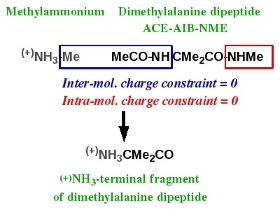Scheme 3

In this new example two Mol_red\$n.p2n files (\$n = 2) corresponding to methylammonium and the N-Acetyl-2-aminoisobutyric_acid-N'-methylamide dipeptide are prepared and uploaded to R.E.D. Server (with or without the corresponding geometry optimization outputs obtained by QM calculations). Two molecular orientations for methylammonium and two conformations (one close to the alpha helix and the other one close to the extended conformation) with four molecular orientations for the dipeptide are used in the charge derivation.
Remarks:
. In the Mol_red1.p2n file provide the keywords describing the inter-molecular charge constraint required for the building of the N-terminal fragment (more generally, the description of inter-molecular charge constraints has to be always provided in the first P2N file of the whole molecule list).
. In the Mol_red2.p2n file provide the keywords describing the intra-molecular charge constraint required for the building of the N-terminal fragment (more generally, the description of intra-molecular charge constraints has to be always provided in the appropriate P2N file).
. Compare the first column of atom names present in the Mol_red1.p2n file used in the charge of the N-Acetyl-2-aminoisobutyric_acid-N'-methylamide dipeptide (section -III.2-) with that used for the N-terminal fragment of this dipeptide.

Downloaded data contain two "Mol_m\$n" directories corresponding to the charge derivation of the two molecules taken individually and a "Mol_MM" directory corresponding to the charge derivation of these molecules taken together. The Tripos mol2 files for the N-terminal fragment of the N-Acetyl-2-aminoisobutyric_acid-N'-methylamide dipeptide are obtained from the "Mol_MM" directory (names: mm\$n-o\$i-FG.mol2, \$n = molecule number, \$i = conformation number; in the present case \$n = \$i = 1 and 2). The following PDF file contains the description of the different files generated by R.E.D. Server for this two-molecules job.

Remark: look at the Cartesian coordinates present in the mm\$n-o\$i-FG.mol2 files, and read the information available here. With the R.E.D. IV June 2010 version the geometry of a fragment originating from the fusion between two molecules is now recalculated.

The RESP-A1A (or "RESP-A1" using R.E.D.-III.x) charge model was used to compute the charge set and to generate the force field library for the N-terminal fragment of the N-Acetyl-2-aminoisobutyric_acid-N'-methylamide dipeptide available in the F-7 R.E.DD.B. project.

-IV.3- (-)OOC-terminal fragment of an amino acid       R.E.D.-III.x compatible

Charge derivation and force field library building for the C-terminal fragment of an amino acid has been discussed in Tutorial -I-. Scheme 4 summarizes the strategy adopted in the AMBER force fields to build such a molecular fragment, taking the dimethylalanine dipeptide as an example. This C-terminal fragment is obtained using two molecules: acetate and the ACE-AIB-NME capped amino acid. Charge derivation for this fragment is carried out by setting to a value of zero two different constraints during the fitting step: (i) an inter-molecular charge constraint between the methyl group of acetate and the CO-NHMe group of atoms of the capped amino acid, and (ii) an intra-molecular charge constraint for the MeCO group of the capped amino acid. Force field library building for this fragment involves removing all the atoms involved in these two constraints, and adding a new atom connectivity between the carboxylate carbon of acetate and the alpha-carbon of the capped amino acid.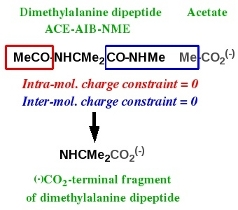Scheme 4

In this new example two Mol_red\$n.p2n files (\$n = 2) corresponding to acetate and the N-Acetyl-2-aminoisobutyric_acid-N'-methylamide dipeptide are prepared and uploaded to R.E.D. Server (with or without the corresponding geometry optimization outputs obtained by QM calculations). Two molecular orientations for acetate and two conformations (one close to the alpha helix and the other one close to the extended conformation) with four molecular orientations for the dipeptide are used in the charge derivation.
Remarks:
. In the Mol_red1.p2n file provide the keywords describing the inter-molecular charge constraint required for the building of the C-terminal fragment (more generally, the description of inter-molecular charge constraints has to be always provided in the first P2N file of the whole molecule list).
. In the Mol_red2.p2n file provide the keywords describing the intra-molecular charge constraint required for the building of the C-terminal fragment (more generally, the description of intra-molecular charge constraints has to be always provided in the appropriate P2N file).
. Compare the first column of atom names present in the Mol_red1.p2n file used in the charge of the N-Acetyl-2-aminoisobutyric_acid-N'-methylamide dipeptide (section -III.2-) with that used for the C-terminal fragment of this dipeptide.

Downloaded data contain two "Mol_m\$n" directories corresponding to the charge derivation of the two molecules taken individually and a "Mol_MM" directory corresponding to the charge derivation of these molecules taken together. The Tripos mol2 files for the C-terminal fragment of the N-Acetyl-2-aminoisobutyric_acid-N'-methylamide dipeptide are obtained from the "Mol_MM" directory (names: mm\$n-o\$i-FG.mol2, \$n = molecule number, \$i = conformation number; in the present case \$n = 2; \$i = 1 and 2). The listing of the files generated during the charge derivation of the C-terminal fragment is exactly the same as that generated for the N-terminal one.

Remark: look at the Cartesian coordinates present in the mm\$n-o\$i-FG.mol2 files, and read the information available here. With the R.E.D. IV June 2010 version the geometry of a fragment originating from the fusion between two molecules is now recalculated.

The RESP-A1A (or "RESP-A1" using R.E.D.-III.x) charge model was used to compute the charge set and to generate the force field library for the C-terminal fragment of the N-Acetyl-2-aminoisobutyric_acid-N'-methylamide dipeptide available in the F-11 R.E.DD.B. project.

-IV.4- Central fragment of a nucleotide       R.E.D.-III.x compatible

Scheme 5 summarizes the strategy adopted in the AMBER force fields to build the central fragment of a nucleotide. This fragment is obtained using two molecules: dimethylphosphate (g, g conformation) and a nucleoside. Charge derivation for this fragment is carried out by setting to a value of zero two inter-molecular charge constraints between the methyl groups of dimethylphosphate and the 5' and 3' hydroxyl groups of the nucleoside. Force field library building for this fragment involves (i) removing all the atoms involved in the two constraints, (ii) adding two atom connectivities between the methoxy oxygens of dimethylphosphate and the C5' and C3' atoms of the nucleoside, and (iii) removing a bond between the phosphorus atom and one of the methoxy oxygens of dimethylphosphate.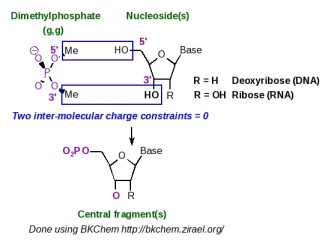Scheme 5

The central fragment of a nucleotide is generated by R.E.D.-III.1. An example of P2N input files useful for the construction of such a nucleotide fragment is available in the R.E.D.-III.1 tools distribution. This molecular fragment is not specifically generated by R.E.D.-IV, and is rather obtained as an element of a set of molecular fragments (see the section -V.3- below in this tutorial).

-IV.5- 5'-terminal fragment of a nucleotide       R.E.D.-III.x incompatible

The 5'-terminal nucleotide fragment is not specifically generated by R.E.D.-IV. It is rather obtained as an element of a set of molecular fragments (see the section -V.3- below in this tutorial).

-IV.6- 3'-terminal fragment of a nucleotide       R.E.D.-III.x incompatible

The 3'-terminal nucleotide fragment is not specifically generated by R.E.D.-IV. It is rather obtained as an element of a set of molecular fragments (see the section -V.3- below in this tutorial).

-IV.7- Molecular fragment of a metal complex       R.E.D.-III.x incompatible

As previously reported, R.E.D. handles charge derivations and force field library building for chemical elements up to Bromine, and does not differentiate a molecule with a metal atom from a molecule without one. For an organo-metallic complex key aspects are the correct definition of the atom connectivities and the spin multiplicity, and a specific approach for the MEP computation and charge fitting step might be required to generate correct charge values. Strategies presented above for the construction of amino acid or a nucleotide fragments can be directly applied for the construction of an organo-metallic complex fragment. The user has simply to set up the correct intra- and/or inter-molecular charge constraints in the Mol_red\$n.p2n input file(s), and R.E.D.-IV will generate the corresponding fragments. Other ideas for defining intra- and inter-molecular charge constraints can be found below.

-V- Force Field Topology DataBase building       R.E.D.-III.x incompatible

-V.1- Definition of a "Force Field Topology DataBase"

A Force Field Topology DataBase (or FFTopDB) regroups an ensemble of force field libraries for different elementary constituents (small molecules and molecular fragments) used to build biopolymers such as proteins, nucleic acids or glycoconjugates. Among many others, examples are the AMBER FFTopDB for nucleic acids and proteins and the GLYCAM FFTopDB for sugars. R.E.D. Server can be used to generate such a FFTopDB in a single R.E.D.-IV execution.

-V.2- Set of amino acid fragments       R.E.D.-III.x incompatible

Scheme 6 represents the simultaneous charge derivation and force field library building of the central, N-terminal and C-terminal fragments of an amino acid taking the dimethylalanine dipeptide as an example. Charge derivation and force field library building for the dipeptide itself is also considered in the approach.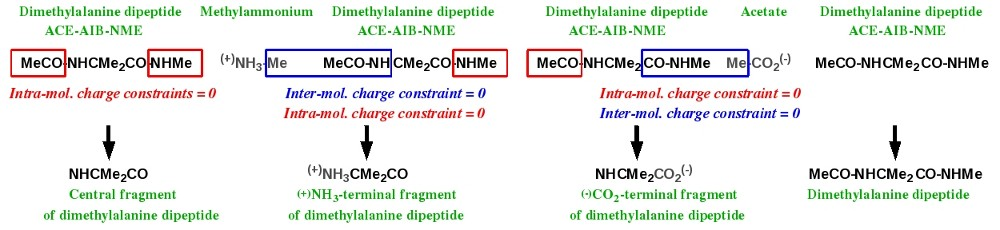Scheme 6

This task can be achieved by juxtaposing the required molecules. Table 4 lists the Mol_red\$n.p2n files (\$n = 6) needed for the simultaneous charge derivation and force field library building for the central, N-terminal and C-terminal fragments of the dimethylalanine dipeptide, as well as for the dipeptide itself.

 Molecule name Dimethylalanine dipeptide Methylammonium Dimethylalanine dipeptide Acetate Dimethylalanine dipeptide Dimethylalanine dipeptide Used for Central frag. N-term. frag. N-term. frag. C-term. frag. C-term. frag. Dipeptide itself P2N files Mol_red1.p2n Mol_red2.p2n Mol_red3.p2n Mol_red4.p2n Mol_red5.p2n Mol_red6.p2n
Table 4

The molecules used in sections -IV.1-, -IV.2- and -IV.3- of this tutorial have to be renumbered and slightly updated before being uploaded to R.E.D. Server.
Remarks:
. Compare the P2N files of the molecules 1, 3, 5 and 6 (more particularly the first column of atom names). Your conclusions ?
. When building a terminal fragment, number the small molecule (methylammonium or acetate) before the dipeptide it is related to in the whole molecule list.
. In the first P2N file of the whole molecule list provide the keywords describing all the inter-molecular charge constraints.
. In the appropriate P2N file provide the keywords describing the intra-molecular charge constraints.

Downloaded data contain six "Mol_m\$n" directories corresponding to the charge derivation of the six molecules taken individually and a "Mol_MM" directory corresponding to the charge derivation of these molecules taken all together. The Tripos mol2 files for the central, N-terminal and C-terminal fragments of the N-Acetyl-2-aminoisobutyric_acid-N'-methylamide dipeptide are obtained from the "Mol_MM" directory (respective names: mm1-o\$i-FG2.mol2, mm3-o\$i-FG.mol2 and mm5-o\$i-FG.mol2; in the present case the conformation number \$i = 1 and 2). The following PDF file contains the description of the different files generated by R.E.D. Server for this six-molecules job.

Remark: look at the Cartesian coordinates present in the mm\$n-o\$i-FG.mol2 files, and read the information available here. With the R.E.D. IV June 2010 version the geometry of a fragment originating from the fusion between two molecules is now recalculated.

R.E.DD.B. contains several projects which follow this approach: the F-74 R.E.DD.B. project is a good example.

Following a slightly more complex approach symbolized in Scheme 7, one could derive charge values and build the force field libraries for the central, N-terminal and C-terminal fragments of more than one amino acid in a single R.E.D.-IV execution. We could even imagine generating a new FFTopDB for the 20 standard residues (i. e. by using 5 * 20 = 100 Mol_red\$n.p2n files) of the AMBER force field plus some additional non-standard ones.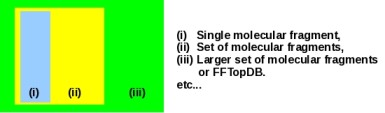Scheme 7

A major update of R.E.DD.B. will be released in a near future. It will include such amino acid FFTopDBs.

Set of amino acid fragments automatically generated from a single dipeptide       R.E.D.-III.x incompatible

A user can automatically derive RESP or ESP charge values, and generate the force field libraries for a (new/modified) dipeptide and its corresponding central, N-terminal and C-terminal amino acid fragments by only providing the P2N file of the considered dipeptide.
Warning: this procedure cannot be applied for proline (and its derivatives) because the amine nitrogen atom of proline is bound to two alkyl groups...

To be able to use this new feature implemented in R.E.D. Server, the following steps have to be followed:

- Generate a PDB file for the dipeptide molecule with the ACE and NME capping groups (i. e. the MeCO and NHMe groups of atoms) being located at the beginning and the end of the PDB file in the atom order, respectively (hydrogen atoms have to be present in the input structure).
- Use this PDB file to generate the corresponding P2N file with R.E.D. Server/Ante_R.E.D. 2.0.
- Check that the ACE and NME capping groups are located at the beginning and the end of the P2N file in the atom order, respectively (one might need to use R.E.D. Server/Ante-R.E.D. 2.0 in its 'non-automatic' mode to keep the input atom order).
- Check/modify the TITLE, CHARGE-VALUE and MULTIPLICITY-VALUE in this P2N file according to your needs.
- Upload this P2N file in R.E.D. Server/R.E.D. IV, and toggle on the "Use RED IV for automatically generating amino acid fragments" option.

Remarks:
. Based on the unique P2N file provided by the user, R.E.D. Server will automatically multiplicate this P2N file, add required keywords (i. e. INTRA-MCC and/or INTER-MCC) in the suitable P2N files, and use internally stored data (P2N and QM output files) related to acetate and methylammonium to generate force field libraries for the dipeptide and its three amino acid fragments.
. When selecting this R.E.D. Server feature, the use of the ACE and NME capping groups is mandatory. Thus, if one wishes to define capping groups others than ACE and NME in the dipeptide P2N file, one has to manually construct the six required P2N files according to Table 4.
. If the Mode 2 of R.E.D. Server/R.E.D. IV is used, a QM geometry optimization output has to be uploaded for the dipeptide molecule with the P2N file.
. Information related to multiple orientation charge fitting is automatically included when generating the P2N file with R.E.D. Server/Ante_R.E.D. 2.0.
. If one wishes to involve multiple conformations in charge derivation the P2N files has to contain the corresponding sets of Cartesian coordinates (See for instance the Mol_red1.p2n file in Table 4).

-V.3- Set of nucleotide fragments       R.E.D.-III.x incompatible

In the AMBER force fields, the central, 5'-terminal and 3'-terminal fragments of a nucleotide are simultaneously generated in a single charge derivation. The strategy for building such nucleotide fragments is summarized in Scheme 8: two inter-molecular charge constraints between the methyl groups of dimethylphosphate and the HO5' and HO3' hydroxyl groups of the nucleoside of interest are used during the fitting step. Following this strategy two different topologies (named as topology A and topology B in Scheme 8), which present the phosphate group located either at the position 5' or 3', respectively, can be obtained. The AMBER force fields arbitrarily chose topology A for nucleic acid construction, and terminal fragments were named 5' and 3' as in regular nucleic acid structures. In R.E.D.-IV, different choices were made: (i) both topologies A and B are generated, and (ii) a more general Y' and X' terminology is used for terminal fragments in order to build natural as well as artificial nucleic acids with various hydroxyl terminal groups.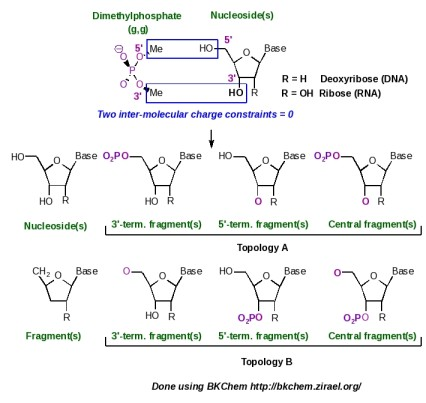Scheme 8

This new example describes the charge derivation and force field library building of the central, 5'-terminal and 3'-terminal nucleotide fragments of adenosine (this ribonucleoside has been already studied in the section -III.3- of this tutorial). Two Mol_red\$n.p2n files (\$n = 2) corresponding to dimethylphosphate (g, g conformation) and to adenosine (Mol_red1.p2n and Mol_red2.p2n) are prepared and uploaded to R.E.D. Server (with or without the corresponding geometry optimization outputs obtained by QM calculations). Four molecular orientations for both molecules were used in the charge derivation.
Remarks:
. When simultaneously building the central, Y'-terminal and X'-terminal fragments for a new nucleotide, number the small molecule used (i. e. dimethylphosphate) before the nucleoside in the whole molecule list.
. In the first P2N file of the whole molecule list provide the keywords describing the two inter-molecular charge constraints.

Downloaded data contain two "Mol_m\$n" directories corresponding to the charge derivation of the two molecules taken individually and a "Mol_MM" directory corresponding to the charge derivation of these molecules taken together. The Tripos mol2 files for the different nucleotide fragments (topologies A and B) are obtained from the "Mol_MM" directory. The following PDF file contains the description of the different files generated by R.E.D. Server for this two-molecules job.
Warning:
. When building regular single or double stranded oligonucleotides, two fragments taken from the topologies A and B should not be mixed.

Following a slightly more complex approach and using additional inter-molecular charge equivalencing (described in Tutorial -I-) between the charges of the deoxyribose atoms belonging to the four regular nucleosides, the ribonucleic acid FFTopDB can be built in a single R.E.D.-IV run. By using the eight regular nucleosides and deoxyribonucleosides, the ribonucleic and deoxyribonucleic acid FFTopDB can be obtained as well.
Remarks:
. Number dimethylphosphate as the first P2N file of the whole molecule list, and provide the keywords describing the inter-molecular charge constraints between dimethylphosphate and the first nucleoside in this first P2N file.
. In the first P2N file of the whole molecule list provide inter-molecular charge equivalencing between the different nucleosides. More information about the implementation of inter-molecular charge equivalencing in R.E.D. IV is available here.
. Look at the Cartesian coordinates present in the Tripos mol2 files generated, and read the information available here. With the R.E.D. IV June 2010 version the geometry of a fragment originating from the fusion between two molecules is now recalculated.

The R.E.DD.B. projects F-45 up to F-56 are examples of such a FFTopDB. In particular, R.E.DD.B. projects F-51 and F-56 illustrate FFTopDBs with a topology B as shown in Scheme 8 with a 3' phosphate connected to a pentose. A major update of R.E.DD.B. will be released in a near future. It will include new FFTopDBs for regular and non-regular nucleic acids.

Set of nucleotide fragments automatically generated from a single nucleoside       R.E.D.-III.x incompatible

A user can automatically derive RESP or ESP charge values, and generate the force field libraries for a (new/modified) nucleoside and its corresponding central, 5'-terminal and 3'-terminal nucleotide fragments by only providing the P2N file of the considered nucleoside.

To be able to use this new feature implemented in R.E.D. Server, the following steps have to be followed:

- Generate a PDB file for the nucleoside molecule, where the four HO5' and HO3' hydroxyl atoms are rigorously named H5T, O5', H3T and O3' (hydrogen atoms have to be present in the input structure).
- Use this PDB file to generate the corresponding P2N file with R.E.D. Server/Ante_R.E.D. 2.0.
- Check that the four HO5' and HO3' hydroxyl atoms bear the names H5T, O5', H3T and O3' in the second column of atom names of the P2N file.
- Check/modify the TITLE, CHARGE-VALUE and MULTIPLICITY-VALUE in this P2N file according to your needs.
- Upload this P2N file in R.E.D. Server/R.E.D. IV, and toggle on the "Use RED IV for automatically generating nucleotide fragments" option.

Remarks:
. Based on the unique P2N file provided by the user, R.E.D. Server will automatically rename this P2N file, add required keywords (i. e. INTRA-MCC and/or INTER-MCC) in the suitable P2N files, and use internally stored data (P2N and QM output files) related to dimethylphosphate to generate force field libraries for the nucleoside and its three nucleotide fragments.
. When selecting this R.E.D. Server feature, the rigorous definition of the H5T, O5', H3T and O3' names in the second column of atom names of the P2N file is mandatory.
. For DNA nucleosides, naming other deoxyribose atoms into C5', H5'1, H5'2, C4', H4', O4', C3', H3', C2', H2'1, H2'2, C1' and H1' in the second column of atom names of the P2N file leads to the use of intra-molecular charge constraints during the fitting step for all the deoxyribose atoms (but the C1' and H1' atoms) in agreement with the Amber force field topology database. This approach is that recommended when one wishes to develop a new force field library for the Cornell et al. Amber force field. A difference in a single atom name prevents the use of intra-molecular charge constraints during the fitting step.
. For RNA nucleosides, naming other ribose atoms into C5', H5'1, H5'2, C4', H4', O4', C3', H3', C2', H2'1, O2', HO'2, C1' and H1' in the second column of atom names of the P2N file leads to the use of intra-molecular charge constraints for all the ribose atoms (but the C1' and H1' atoms) in agreement with the Amber force field topology database. This approach is that recommended when one wishes to develop a new force field library for the Cornell et al. Amber force field. A difference in a single atom name prevents the use of intra-molecular charge constraints during the fitting step.
. For chemically engineered and articial nucleosides with a modified monosaccharide, no intra-molecular charge constraint is added during the charge fitting step.
. If the Mode 2 of R.E.D. Server/R.E.D. IV is used, a QM geometry optimization output has to be uploaded for the nucleoside molecule with the P2N file.
. Information related to multiple orientation charge fitting is automatically included when generating the P2N file with R.E.D. Server/Ante_R.E.D. 2.0.
. If one wishes to involve multiple conformations in charge derivation the P2N files has to contain the corresponding sets of Cartesian coordinates.

-V.4- Set of glycoconjugate fragments       R.E.D.-III.x incompatible

This example is taken from the work published in J. Org. Chem. 2007, 72, 9032-9045 by Gouin et al. Because of the absence of triazole fragments in the GLYCAM force field, a new FFTopDB for the different glycoclusters described in Scheme 9 has been developed. In this work, five molecules (each one represented by two conformations and four molecular orientations) were involved in the charge derivation, and eight inter-molecular charge constraints and one intra-molecular charge constraint were used in the fitting step to define the connections between the different units.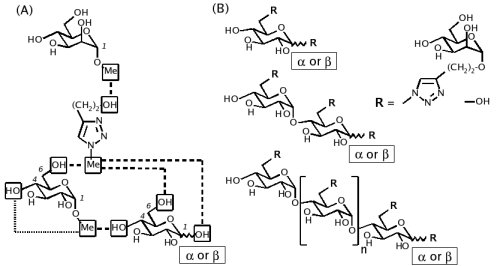(A) FFTopDB built using four monosaccharides & a triazole derivative; (B) Construction of various glycoclusters based the FFTopDB previously defined.
Dashed line: inter-molecular charge constraints. Ultra-fine dashed line: intra-molecular charge constraint.

Scheme 9

Table 5 lists the Mol_red\$n.p2n files (\$n = 5) needed for the charge derivation and force field library building of the glycoclusters reported in Scheme 9.

 α-O-methyl-Mannoside Triazol-linker α-O-methyl-Glucoside α-D-Glucose β-D-Glucose Mol_red1.p2n Mol_red2.p2n Mol_red3.p2n Mol_red4.p2n Mol_red5.p2n
Table 5

The RESP-C2 charge model was used to compute the charge set and to generate the glycocluster FFTopDB available in the F-71 R.E.DD.B. project. The script allowing the use of these force field libraries and the construction of the glycoconjugates in the LEaP program is also available in this R.E.DD.B. project. Finally, the F-84 R.E.DD.B. project has been submitted, and represents a direct extension of the "F-71" R.E.DD.B. project.

-VI- All together in a single R.E.D.-IV run?       R.E.D.-III.x incompatible

We could finally imagine the simultaneous generation of different FFTopDBs for amino acids, nucleotides and monosaccharides in a single R.E.D.-IV execution (Scheme 10). In principle, there is no limit to the strategy of juxtaposing P2N input files in the R.E.D.-IV approach. However, to be successful the user has to follow some important rules:

- The RESP program must undergo some modifications (qtol = 0.1d-5, maxq = 5000, maxlgr = 500 and maxmol = 200 parameters), and has to be recompiled.
- An intra-molecular charge constraint is always defined in the Mol-red\$n.p2n file it is related to.
- An inter-molecular charge constraint is always defined in the first Mol-red\$n.p2n file of the whole molecule list.
- Inter-molecular charge equivalencing is always defined in the first Mol-red\$n.p2n file of the whole molecule list.
- The simultaneous charge derivation and force field library building for different FFTopDBs is only possible if the same algorithms are used in the charge derivation for the different molecules. An example of limitation is the use of the Connolly surface and CHELPG algorithms in the molecular electrostatic potential computations for AMBER and GLYCAM force fields, respectively.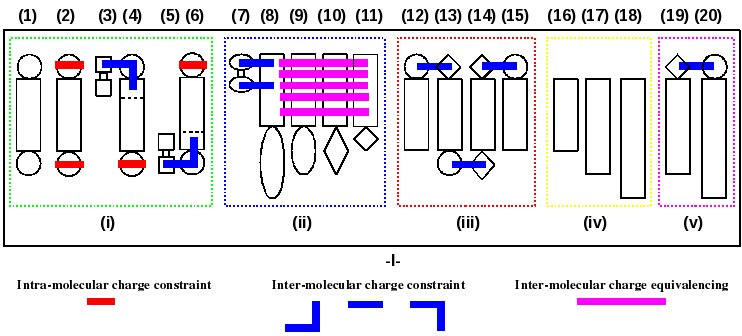Scheme 10

(1): a dipeptide "A",
(2): the central fragment of "A",
(3-4): the N-terminal fragment of "A" using methylammonium (3) and "A" (4),
(5-6): the C-terminal fragment of "A" using acetate (5) and "A" (6),
(7-11): a nucleic acid FFTopDB using dimethylphosphate (7) and 4 or more nucleosides "B", "C", "D" & "E",
(12-15): a glycoconjugate constituted of 4 or more different building blocks "F", "G", "H" & "I",
(16-18): 3 or more indendent ligands "J", "K" & "L" of a receptor,
(19-20): an organo-metallic complex based on 2 building blocks "M" & "N",
(i): FFTopDB of new amino acids (the number of amino acids being not limited to 1),
(ii): FFTopDB of a set of modified nucleotides,
(iii): FFTopDB for a glycoconjugate,
(iv): FFTopDB for a set of receptor ligands,
(v): FFTopDB for an organic-metallic complex.
-I-: Charge derivation and force field library building involving 20 molecules (each of them being represented by a different numbers of molecular conformations and orientations) executed in a single R.E.D.-IV run.

Should you find any mistake in this tutorial, please, send me an e-mail: